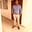a concise shot of dev knowledge
Become a Contributor
Concise shots of dev knowledge

RELATED TAGS

numpy
communitycreator

# How to mask an array outside a given interval in NumPyOnyejiaku Theophilus Chidalu

## Overview

In NumPy, the masked_outside() function is used to mask an array outside a given interval.

### Syntax

ma.masked_outside(x, v1, v2)

### Parameters

This function takes the following parameter values:

• x: This is the input array.
• v1 and v2 : These represent the boundary for which the values of the given array are not to be masked. Both values—v1 and v2—are not included when masking.

### Return value

This function returns a masked array with values within the specified intervals masked.

### Example

# A code to illustrate the masked_inside() function

# importing the necessary libraries
import numpy as np
import numpy.ma as ma

# creating an input array
my_array = np.array([1, 2, 3, 4, 5, 6, 7, 8, 9, 10])

# masking the values outside 3 to 7

print(mask_array)

### Explanation

• Line 4–5: We import the necessary library and module.
• Line 8: We create an input array, my_array.
• Line 11: We mask the values outside the interval of 37 in the input array using the masked_outside() function. The result is assigned to a variable, mask_array.
• Line 13: We print the value of the masked array, mask_array.

RELATED TAGS

numpy
communitycreator

CREATOROnyejiaku Theophilus Chidalu
RELATED COURSES

View all Courses

Keep Exploring

Learn in-demand tech skills in half the time